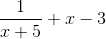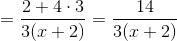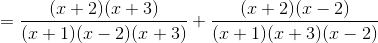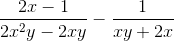# Add, Subtract, Simplify Rational Expressions Examples With Solutions

How to add, subtract and simplify Rational Expressions? Grade 11 examples are presented along with detailed solutions and more questions with detailed Solutions and explanations are included. We first start with adding, subtracting and simplifying fractions and then we move on to rational expressions.

An online calculator to simplify rational expressions is included and may be used to check results.

## How to add, subtract and simplify rational expressions?

Adding, subtracting and simplifying rational expressions is done in the same way as adding, subtracting and simplifying fractions. Two cases are possible:
case 1: The fractions or rational expressions have the same denominator, we therefore add or subtract as follows:

\dfrac{A}{B} \pm \dfrac{C}{B} = \dfrac{A\pm C}{B}
case 2: The fractions or rational expressions do not have the same denominator, we first convert to a common denominator then add or subtract.

In fractions, integers only are involved while algebraic expressions are involved in rational expressions.

If you have difficulties in adding, subtracting and simplifying fractions and rational expressions, this tutorial will help you overcome those difficulties on the condition that you understand every step involved in solving these questions and also spend more time practicing if needed. I will present the examples below starting with fractions first and then with rational expressions, with more challenging questions as you walk through the tutorial. You need to understand each step!

### Example 1

Subtract and simplify:
\dfrac{2}{3} - \dfrac{4}{3}
Solution:
The two fractions have the same denominator and therefore we subtract as follows
\dfrac{2}{3} - \dfrac{4}{3} = \dfrac{2-4}{3} = - \dfrac{2}{3}

### Example 2

Subtract and simplify:
\dfrac{1}{5} - \dfrac{7}{10}
Solution:
The two fractions have different denominators and we therefore need to convert them to the same denominator.
We first find the lowest common multiple (LCM) of the two denominators 5 and 10.

5: 5,
10 , 15, ... (multiply 5 by 1, 2, 3, ... to obtain a list of multiples of 5)
10:
10 , 20, 30, ... (multiply 10 by 1, 2, 3, ... to obtain a list of multiples of 10)
The first common multiple (or the lowest, in red in the lists above) will be used as the common denominator which is also called lowest common denominator (LCD).
We now convert all denominator to the common denominator 10 as follows:
\dfrac{1}{5} - \dfrac{7}{10} = \dfrac{1 \times \color{red}{2}}{5 \times \color{red}{2}} - \dfrac{7}{10} = \dfrac{2}{10} - \dfrac{7}{10}
then simplify
= \dfrac{2-7}{10} = - \dfrac{5}{10} = - \dfrac{1}{2}

### Example 3

Simplify:
\dfrac{5}{8} + \dfrac{1}{12} - \dfrac{5}{16}
Solution:
The three denominators are different and therefore we need to find a common denominator.
We first find the lowest common multiple (LCM) of the two denominators 8, 12 and 16.

8: 8, 16, 24, 32, 40,
48 , 56, 64, 72, 80,...
12: 12, 24, 36,
48 , 60, 72, 84, 96,...
16: 16, 32,
48 , 64, 80, 96...
The lowest common denominator is 48 and we now convert all 3 denominators to the common denominator 48.
\dfrac{5}{8} + \dfrac{1}{12} - \dfrac{5}{16} = \dfrac{5 \times \color{red}{6}}{8 \times \color{red}{6}} + \dfrac{1 \times \color{red}{4}}{12 \times \color{red}{4}} - \dfrac{5 \times \color{red}{3}}{16 \times \color{red}{3}}
and then simplify as follows:
= \dfrac{30}{48} + \dfrac{4}{48} - \dfrac{15}{48} = \dfrac{30+4-15}{48} = \dfrac{19}{48}

### Example 4

Subtract and simplify:
\dfrac{2-x}{x+2} - \dfrac{4}{x+2}
Solution:
The two rational expressions have the same denominator and therefore we subtract as follows
\dfrac{2-x}{x+2} - \dfrac{4}{x+2} = \dfrac{2-x-4}{x+2}
then simplify
\dfrac{-x-2}{x+2} = \dfrac{-\cancel{(x+2)}}{\cancel{x+2}} = - 1 , \text {for } x \ne -2

### Example 5

Write as a rational expression:.
Solution:
In order to add a rational expression with an expression without denominator, we convert the one without denominator into a rational expression then add them.The two rational expressions have the same denominator and they are added as follows:Expand the product (x-3)(x+5) and simplify.NOTE: For the following examples, you need to know How to Find lowest common multiple (LCM) of Expressions and also practice on questions on detailed solutions on LCM .

### Example 6

Add and simplify:.
Solution:
The two rational expressions have different denominators. In order to add the rational expressions above, we need to convert them to a common denominator. We first factor completely the two denominators 3x + 6 and x + 2 and find the LCM.
3 x + 6 = 3(x + 2)
x + 2 = x + 2
LCM = 3(x + 2)
We now use the LCM as the common denominator and rewrite the rational expressions with the same denominator as follows.### Example 7

Add and simplify:.
Solution:
The two rational expressions have different denominators. In order to add the rational expressions above, we need to convert them to a common denominator. We factor completely the two denominators 3x - 3 and 2 x + 4 and find the LCM.
3 x - 3 = 3(x - 1)
2 x + 4 = 2(x + 2)
LCM = 3 � 2 (x - 1)(x + 2) = 6(x - 1)(x + 2)
We now use the LCM as the common denominator and rewrite the rational expressions with the same denominator as follows.We now add, expand the terms in the numerator and simplify.### Example 8

Add and simplify:.
Solution:
The two rational expressions have different denominators. In order to add the rational expressions above, we need to convert them to a common denominator. We first factor completely the two denominators x 2 - x - 2 and x 2 + 4 x + 3 and find the LCM.
x
2 - x - 2 = (x + 1)(x - 2)
x
2 + 4 x + 3 = (x + 1)(x + 3)
LCM = (x + 1)(x - 2)(x + 3)
We now use the LCM as the common denominator and rewrite the rational expressions with the same denominator as follows.We now add the numerators, factor x + 2 and simplify.The numerator in factored form is very useful in many situations: solving rational inequalities, solving rational equations, graphing rational functions, ....

### Example 9

Subtract and simplify:.
Solution:
The two rational expressions have different denominators. In order to add the rational expressions above, we need to convert them to a common denominator. We factor completely the two denominators x 2 + 6 x + 5 and x 2 + 11 x + 30 and find the LCD.
x
2 + 6 x + 5 = (x + 1)(x + 5)
x
2 + 11 x + 30 = (x + 6)(x + 5)
LCD = (x + 1)(x + 5)(x + 6)
We also factor the numerators.
x
2 + 3 x + 2 = (x + 1)(x + 2)
x
2 + 4 x - 12 = (x + 6)(x - 2)
We rewrite the given expression with numerators and denominators in factored form and simplify if possible.### Example 10

Simplify:.
Solution:
The three rational expressions have different denominators. In order to subtract/add the rational expressions above, we need to convert them to a common denominator.List and factor completely the three denominators x + 3 , x 2 + x - 6 and x - 2 and find the LCM.
x + 3 = x + 3
x
2 + x - 6 = (x + 3)(x - 2)
x - 2 = x - 2
LCM = (x + 3)(x - 2)
We now use the LCM as the common denominator and rewrite the rational expressions with the same denominator as follows.We now add the numerators and simplify.### Example 11

Subtract and simplify:.
Solution:
The two rational expressions have different denominators. In order to subtract/add the rational expressions above, we need to convert them to a common denominator. List and factor completely the two denominators 2 x 2 y - 2 x y and x y + 2 x and find the LCM.
2 x
2 y - 2 x y = 2 x y (x - 1)
x y + 2 x = x(y + 2)
LCM = 2 x y (x - 1)(y + 2)
We now use the LCM as the common denominator and rewrite the rational expressions with the same denominator as follows.Expand and simplify.### More Questions: Add, subtract and simplify the following:

Detailed
Solutions and explanations to these questions.Detailed
Solutions and explanations to these questions.